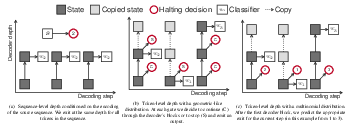EN FR
EN FR

## Section: New Results

### Pluri-disciplinary Research

#### Biological Sequence Modeling with Convolutional Kernel Networks

Participants : Dexiong Chen, Laurent Jacob, Julien Mairal.

The growing number of annotated biological sequences available makes it possible to learn genotype-phenotype relationships from data with increasingly high accuracy. When large quan- tities of labeled samples are available for training a model, convolutional neural networks can be used to predict the phenotype of unannotated sequences with good accuracy. Unfortunately, their performance with medium- or small-scale datasets is mitigated, which requires inventing new data-efficient approaches. In this paper , , we introduce a hybrid approach between convolutional neural networks and kernel methods to model biological sequences. Our method, shown in Figure 17, enjoys the ability of convolutional neural networks to learn data representations that are adapted to a specific task, while the kernel point of view yields algorithms that perform significantly better when the amount of training data is small. We illustrate these advantages for transcription factor binding prediction and protein homology detection, and we demonstrate that our model is also simple to interpret, which is crucial for discovering predictive motifs in sequences. The source code is freely available at https://gitlab.inria.fr/dchen/CKN-seq.

Figure 17. Construction of single-layer (left) and multilayer (middle) CKN-seq and the approximation of one layer (right). For a single-layer model, each $k$-mer ${P}_{i}\left(𝐱\right)$ is mapped to ${\varphi }_{0}\left({P}_{i}\left(𝐱\right)\right)$ in $ℱ$ and projected to $\Pi {\varphi }_{0}\left({P}_{i}\left(𝐱\right)\right)$ parametrized by ${\psi }_{0}\left({P}_{i}\left(𝐱\right)\right)$. Then, the final finite-dimensional sequence is obtained by the global pooling, $\psi \left(𝐱\right)=\frac{1}{m}{\sum }_{i=0}^{m}{\psi }_{0}\left({P}_{i}\left(𝐱\right)\right)$. The multilayer construction is similar, but relies on intermediate maps, obtained by local pooling.#### Recurrent Kernel Networks

Participants : Dexiong Chen, Laurent Jacob [CNRS, LBBE Laboratory] , Julien Mairal.

Substring kernels are classical tools for representing biological sequences or text. However, when large amounts of annotated data are available, models that allow end-to-end training such as neural networks are often preferred. Links between recurrent neural networks (RNNs) and substring kernels have recently been drawn, by formally showing that RNNs with specific activation functions were points in a reproducing kernel Hilbert space (RKHS). In this paper , we revisit this link by generalizing convolutional kernel networks—–originally related to a relaxation of the mismatch kernel—–to model gaps in sequences. It results in a new type of recurrent neural network (Figure 18), which can be trained end-to-end with backpropagation, or without supervision by using kernel approximation techniques. We experimentally show that our approach is well suited to biological sequences, where it outperforms existing methods for protein classification tasks.

Figure 18. Representation of a sequence in a RKHS based on our kernel.